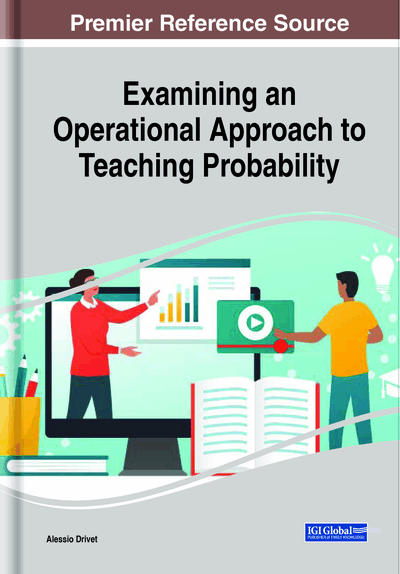# Classical Probability

DOI: 10.4018/978-1-7998-3871-5.ch001
Available
\$29.50
No Current Special Offers

## Abstract

Classical probability is historically presented as the first interpretation of random phenomena. Even with its application limits, it represents the starting point for the main concepts of probability calculation. In class it is the most represented formulation, and, in some cases, it is presented as a standard definition of probability. In the chapter, after presenting the classic objects (coins, marbles, dice, playing cards) normally used to introduce the theme, the panorama is widened thanks to the introduction of less common artifacts and the use of examples that can provide useful insights for further study. The path starts from the concepts of trial and event and ends with the Bayes theorem.
Chapter Preview
Top

## Introduction

Anytime you have a 50–50 chance of getting something right, there’s a 90 percent probability you’ll get it wrong. ~ Andy Rooney

To understand a discipline it is essential to follow its historical developments. This is especially true for probability; it has a particularly complex development, especially in the most ancient phases. The chance was connected with mystical and religious aspects and it will take a long time before the subject is tackled rationally and takes its “mathematical” form. Suffice it to recall the case of Bishop Wibold who, just before the end of the first millennium, enumerated 56 theological virtues, each corresponding to the possible ways in which three dice could be thrown and therefore chose a particular virtue to meditate in the next 24 hours. For more on the historical aspects, see Kendall.

Historically we perhaps owe to Gerolamo Cardano (1501-1576) the first writing on probability; he, in the book Liber de ludo aleæ, collects the observations made during his career as a fierce player and calculates the probability of the results obtained by throwing three dice.

This is no accident, in the sense that the first thoughts on the subject arise from the need to find rules in gambling. The problem of the distribution of the stakes was addressed by Luca Pacioli (1445-1517) in the Summa de arithmetica, geometry, proportioni et proportionality and later by Niccolò Tartaglia (1499-1557); they deal with the problem of how to distribute the stakes in case the game should be interrupted. This theme was later resolved by Blaise Pascal (1623-1662) and Pierre de Fermat (1601-1665). The story of the collaboration of the latter two great characters in solving another type of problem is extremely interesting. What is it about? A fashionable game at that time was the following: the game manager would bet at par with a player that the latter, by rolling a dice 4 times, would get the number 6 at least once. A fierce player like Antoine Gombaud, Cavalier de Méré had always thought that getting at least a 6 in 4 throws of a dice was equivalent to getting at least a double 6 in 24 rolls. However, playing according to that belief, he lost and wrote to Pascal complaining that the math was failing. Pascal and Fermat solved the problem by demonstrating that it was not mathematics that failed but the knight's erroneous reasoning. The problem is what is traditionally associated with the birth (1654) of probability calculation as a scientific discipline.

After various progresses, with J. Bernoulli (1654-1705), Abraham De Moivre (1667-1754) and Pierre-Simon Laplace (1749-1827) the classical definition of probability is crystallized as the ratio between favorable cases and possible cases supposed all equally possible.

The following sentence is due to Laplace: “The theory of chance consists in reducing all the events of the same kind to a certain number of cases equally possible” as reported by Dhombres.

The classical definition has the advantage of being simple and operational, but it has a negative aspect: in fact, the concept of probability is introduced by exploiting the notion of comparable events which in turn implies the knowledge of the idea of probability. The result is an evident vicious circle. Laplace himself realized this and tried to overcome the problem by introducing the “principle of sufficient reason” according to which two events are to be considered comparable when there are no valid reasons to think that one of them can occur more easily than the other.

This severely limits the scope because in reality this condition cannot always be had. As a consequence, we can say that the classical probability is applicable in all cases in which for reasons of symmetry we can think equally possible all cases: tossing coins or dice not rigged, drawing cards from a deck or balls from an urn, output of bingo numbers, etc..

Top

## Coins

Before starting the topic it is nice to start from a story, from which it is possible to develop some reasoning.

## Key Terms in this Chapter

Sample Space: All the possible outcomes of an experiment.

Swiss Cheese Model: Model used in risk analysis and risk management.

Trial: An experimental procedure.

Confusion of the Inverse: Is the logical fallacy when a conditional probability is exchanged for its inverse.

Impossible Event: Random event that defines the empty set.

Law of Conditional Probabilities: A measure of the probability of an event occurring given that another event has occurred.

Parameters: Values characterizing a particular model.

Events: A set of outcomes of an experiment.

Law of Truly Large Numbers: With a large enough number of samples, anything is likely to be observed.

Certain Event: Random event that defines the entire sample space.

Law of Compound Probability: Term relating to the probability of two independent events occurring.

Opposite Event: Given an event, the opposite event happens when the first did not occur.

Law of Total Probability: It expresses the total probability of an outcome.

Opposite Probability: The probability of an opposite event.

Random Variable: A variable whose values depend on outcomes of a random phenomenon.

## Complete Chapter List

Search this Book:
Reset